# Static Program Analysis学习笔记

78

### IR(Intermediate Representation)

#### AST vs IR(Why IR?)

AST:

high-level and closed to grammar structure

usually language dependent

suitable for fast type checking

lack of control flow information

IR:

low-level and closed to machine code

usually language independent

compact and uniform

contains control flow information

usually considered as the basis for static analysis

#### Jimple Basic Concepts:

The four main method calls of JVM:

invokespecial: call constructor, call superclass methods, call private methods

invokevirutal: instance methods call (virtual dispatch)

invokeinterface: cannot optimization, checking interface implementation

invokestatic: call static methods

method signature: `class name:return type method_name(parameter1 type,paramter 2 type...)`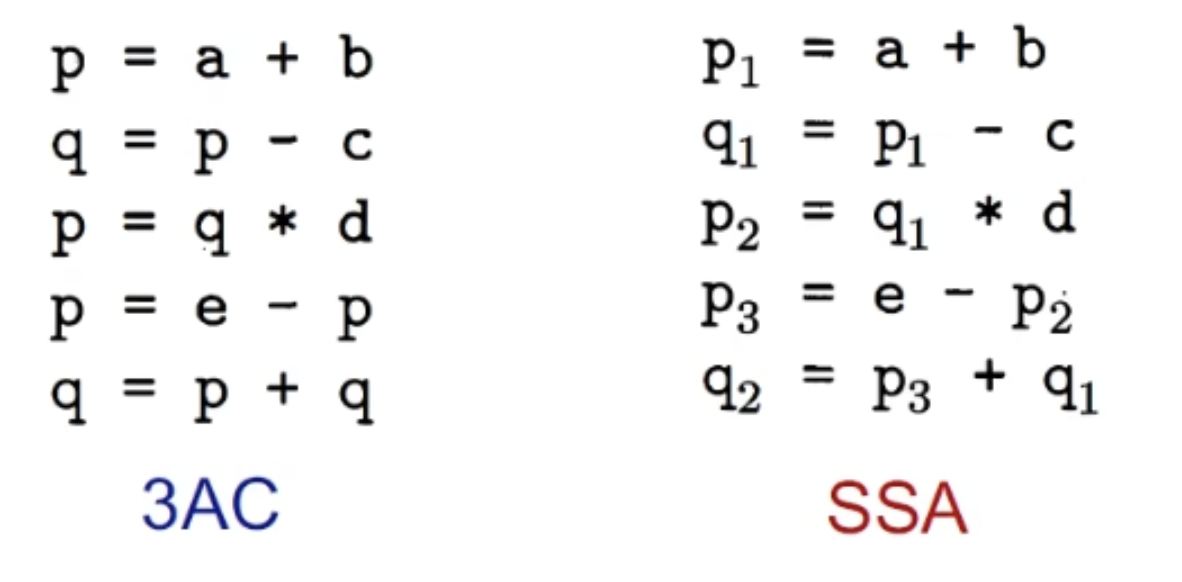3AC:

There is at most one operator on the right side of an instruction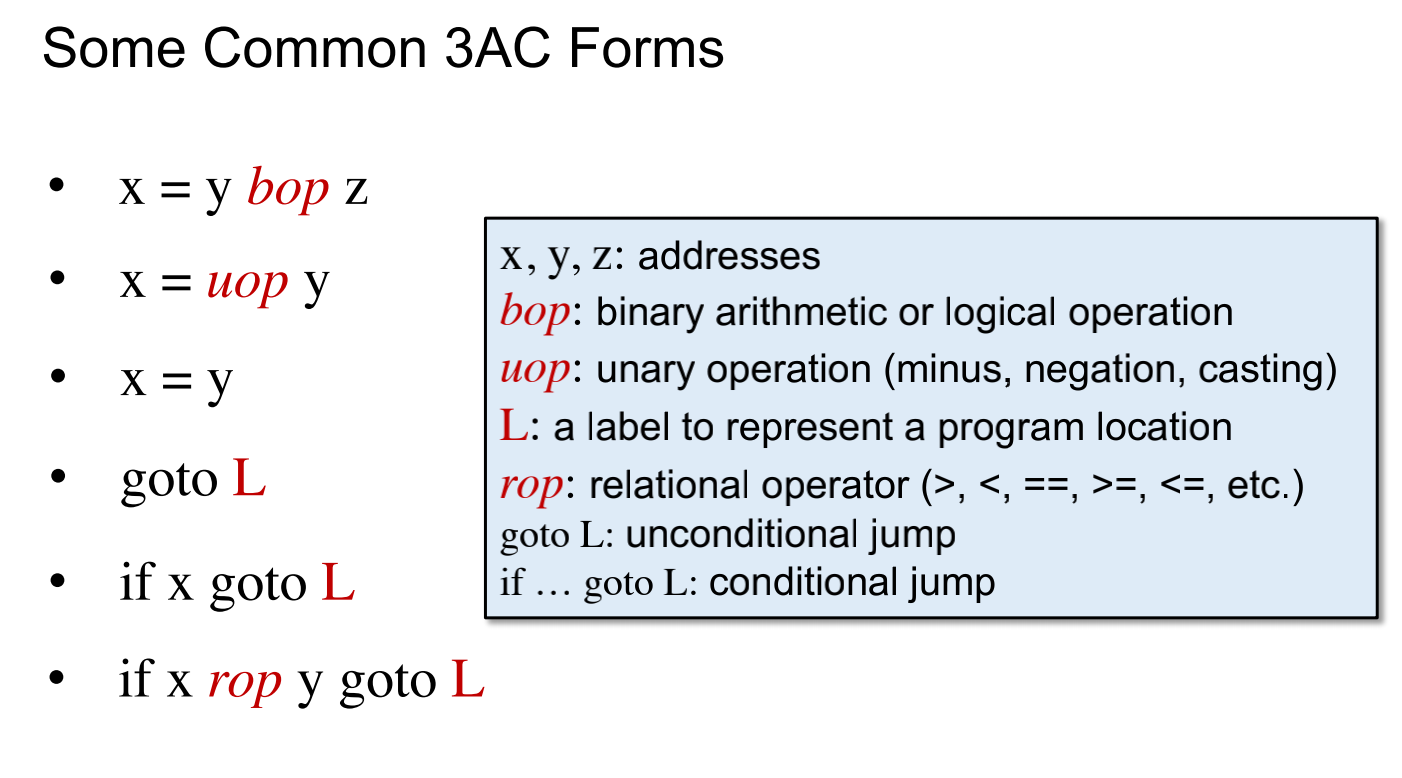In 3AC, special control statements (such as GOTO and IF statements) are used to describe the program's control flow

SSA:

Every variable has exactlly one definitiontion

in SSA, the program's control flow is represented using basic blocks and phi functions. The phi function is defined at the entry of a basic block and is used to merge different variable values into one value to handle the basic block's control flow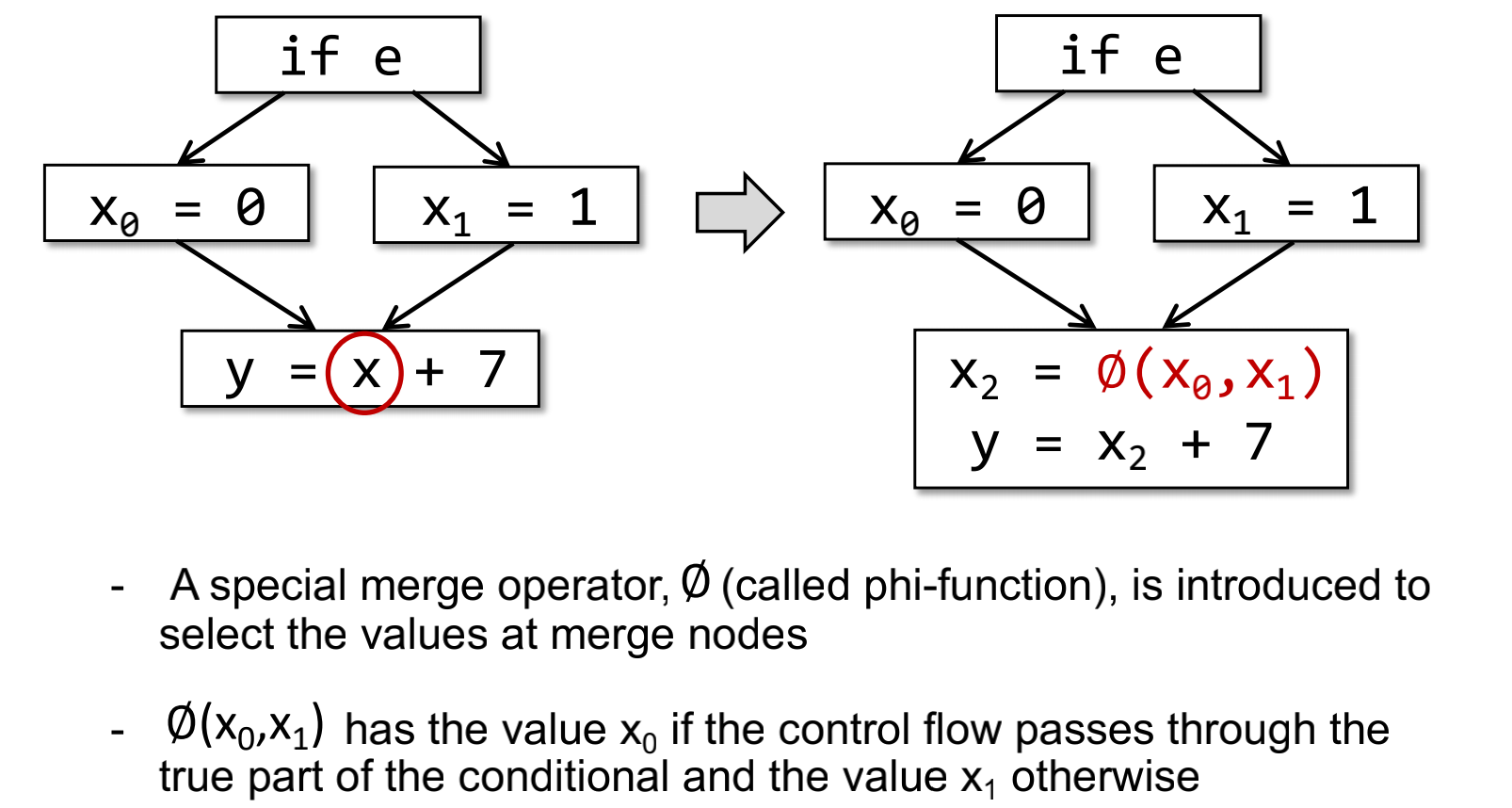#### Basic Blocks(BB)

maximal sequences of consecutive three-address instructions with the properties that It can be entered only at the beginning and it can be exited only at the end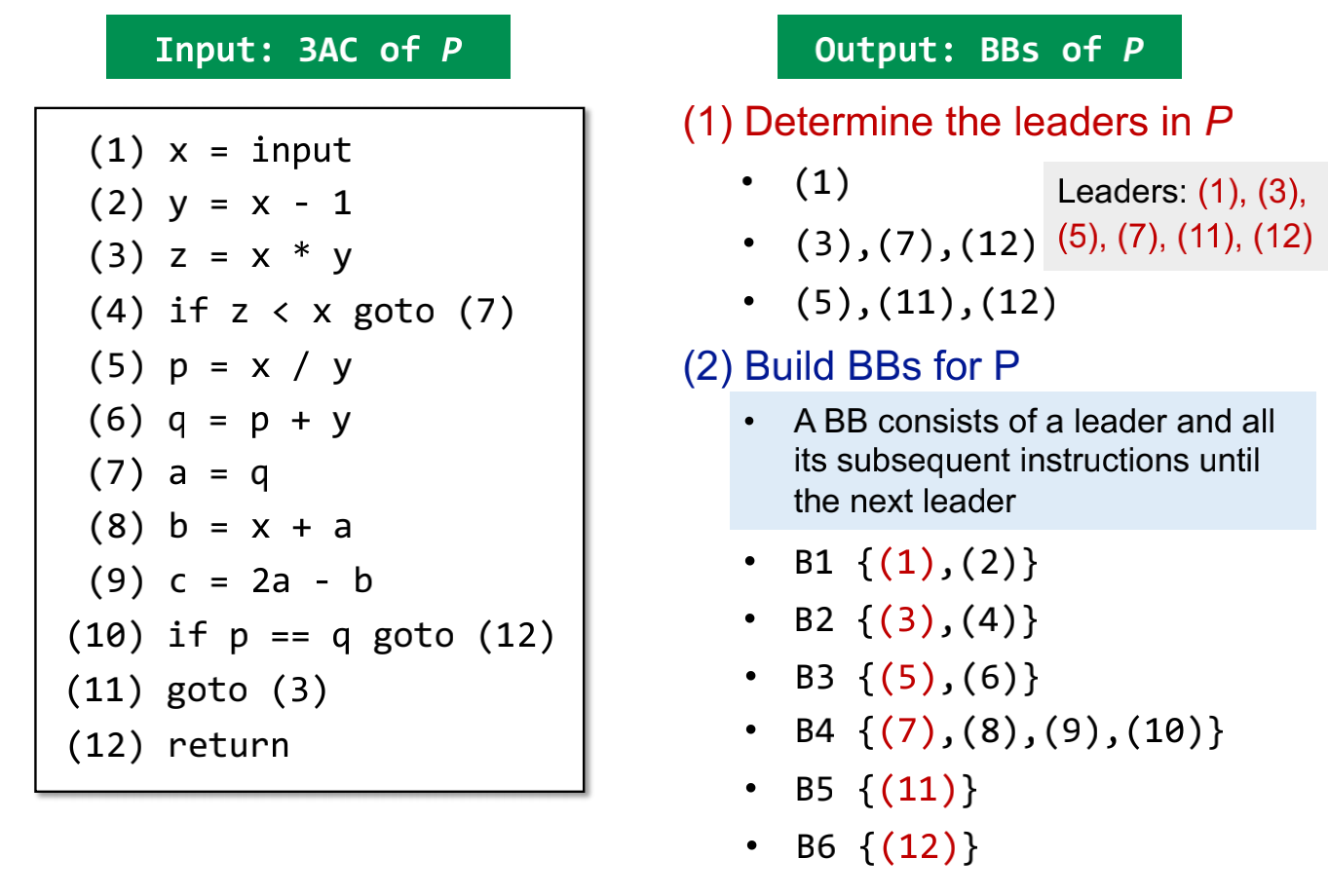#### Control Flow Graph (CFG)

The nodes of CFG are basic blocks

Build CFG: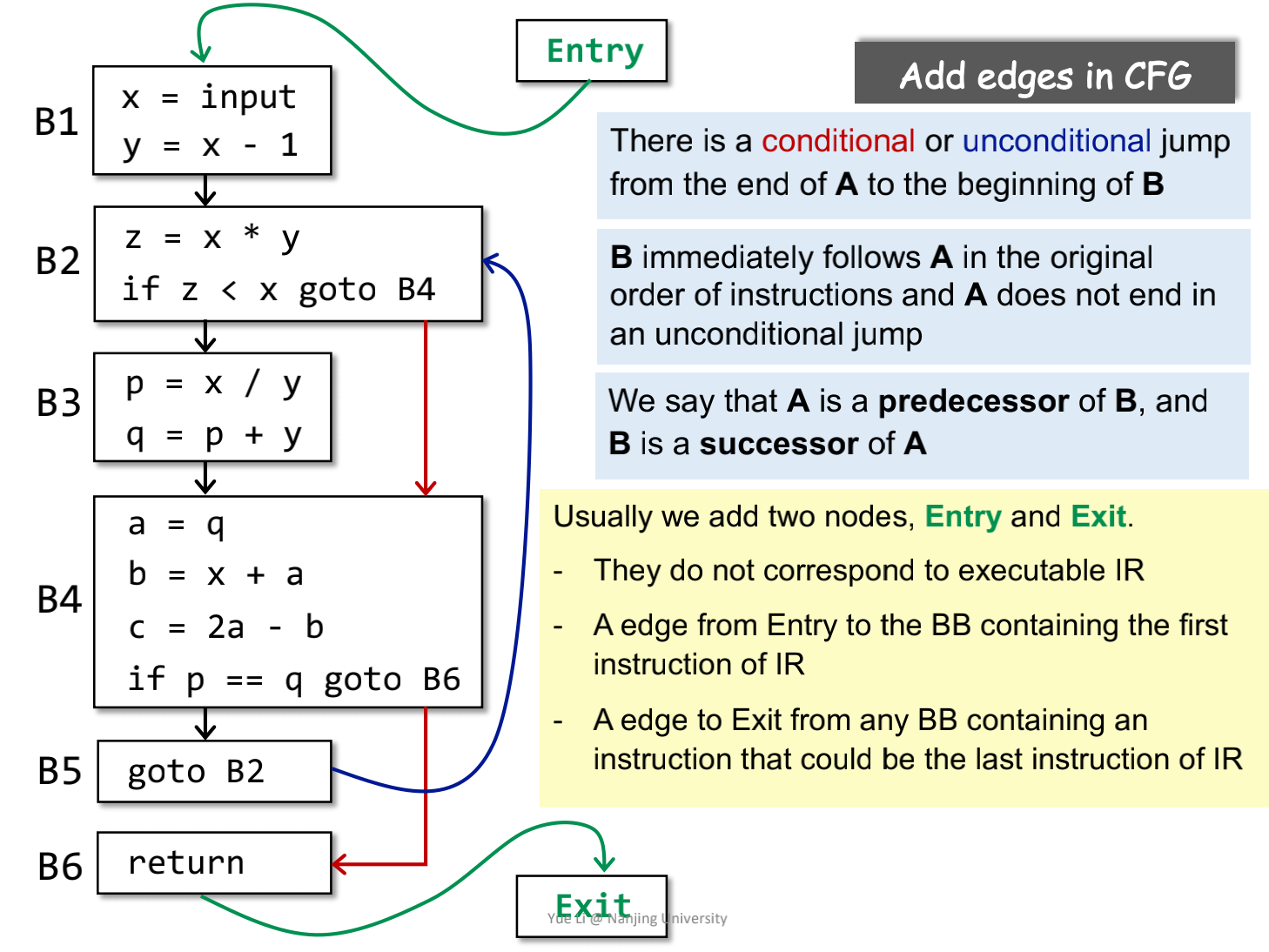### Data Flow Analysis

#### overview

How Data Flows on CFG?

Flows throw the Nodes(BBs/statements) and Edges(control flows) of CFG(program)

How application-specific Data <- Abstraction

may analysis(for most static analyses):

outputs information that may be true (over-approximation)

must analysis:

outputs information that must be true (under-approximation)

Over- and under-approximations are both for safety of analysis

#### Applications

##### Reaching Definitions Analysis

A definition d at program point p reaches a point q if there is a path from p to q such that d is "not killed" along the path

Safe-approximation

Algorithm: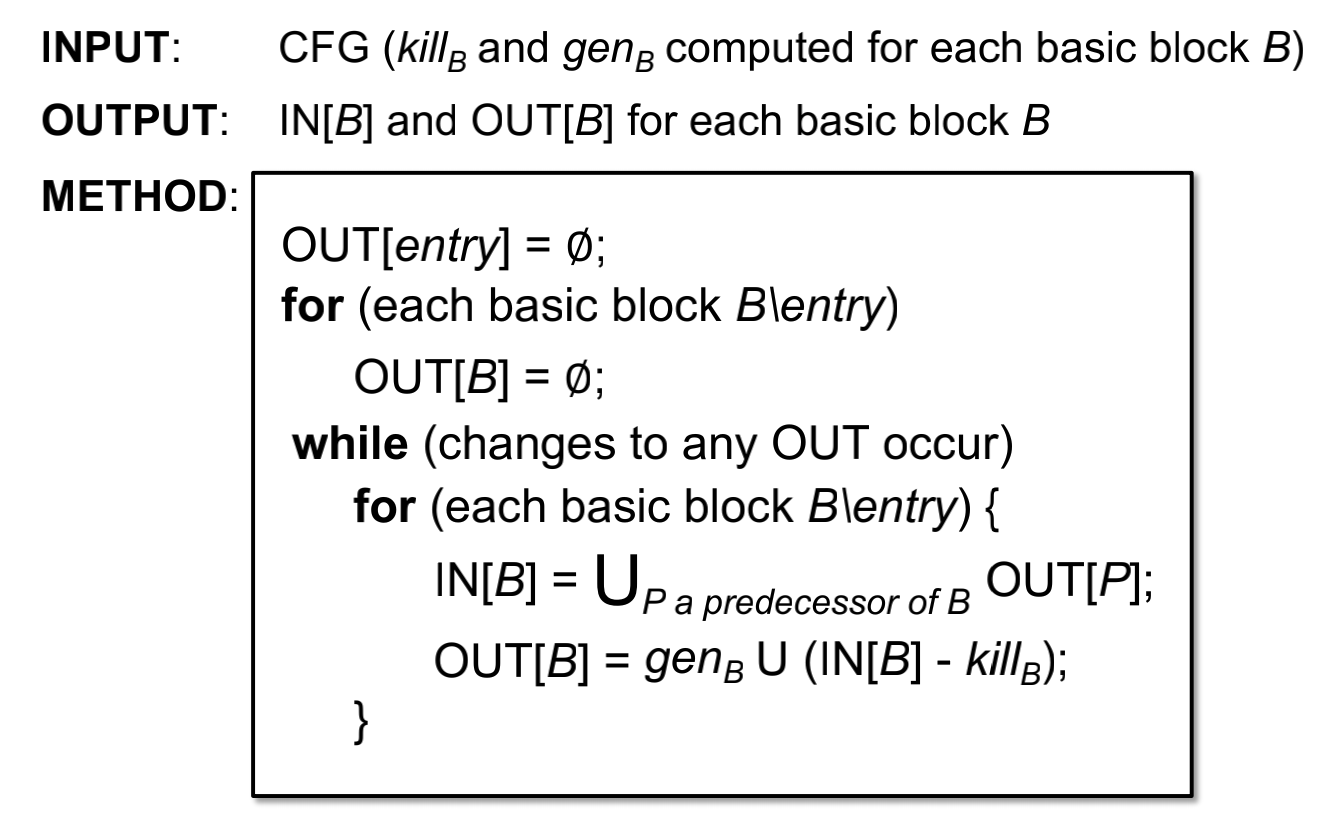Example: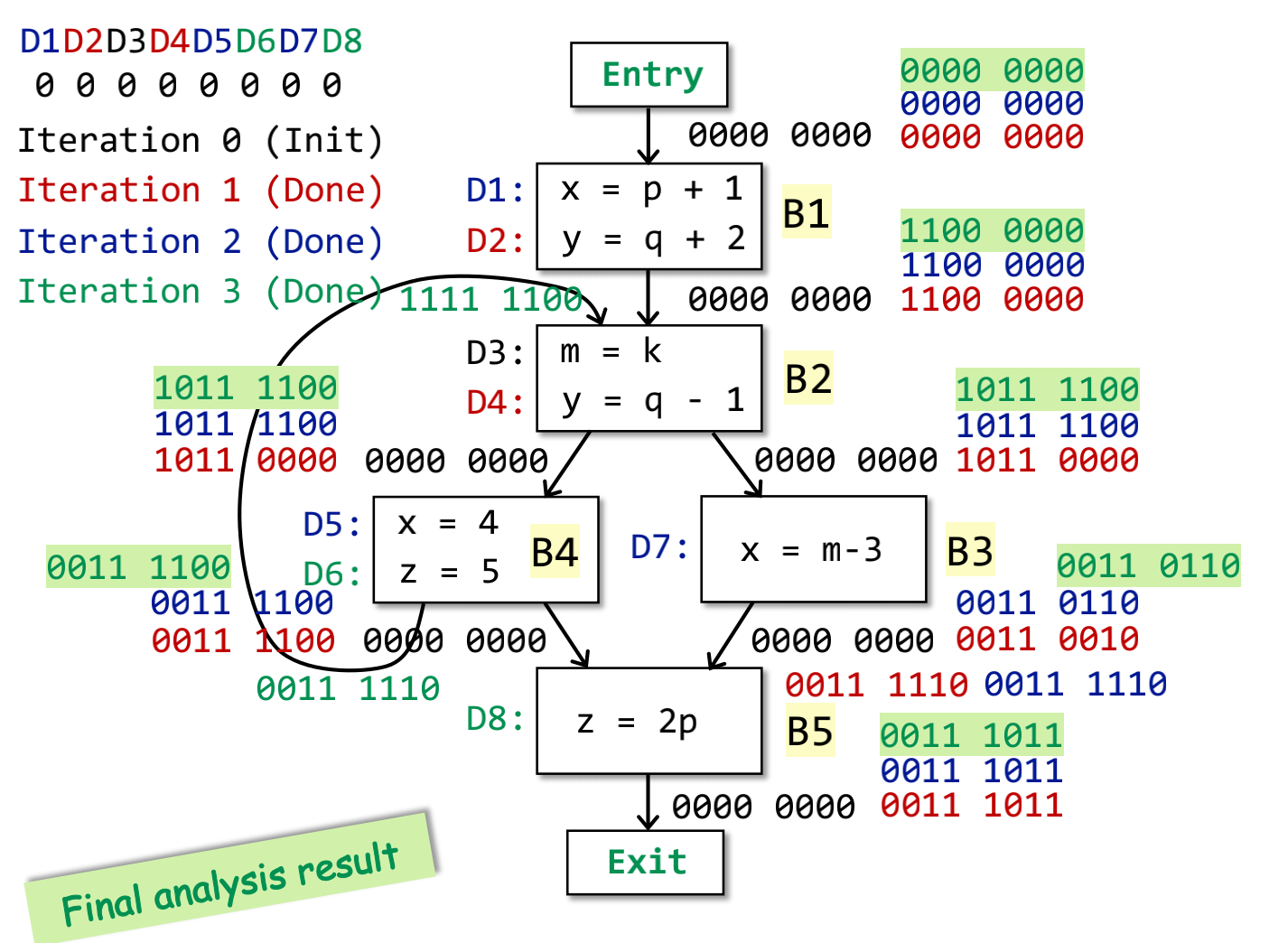##### Live Variables Analysis

Live variables analysis tells whether the value of variable v at program point p could be used along some path in CFG starting at p.If so, v is live at p; otherwise, v is dead at p.

Algorithm: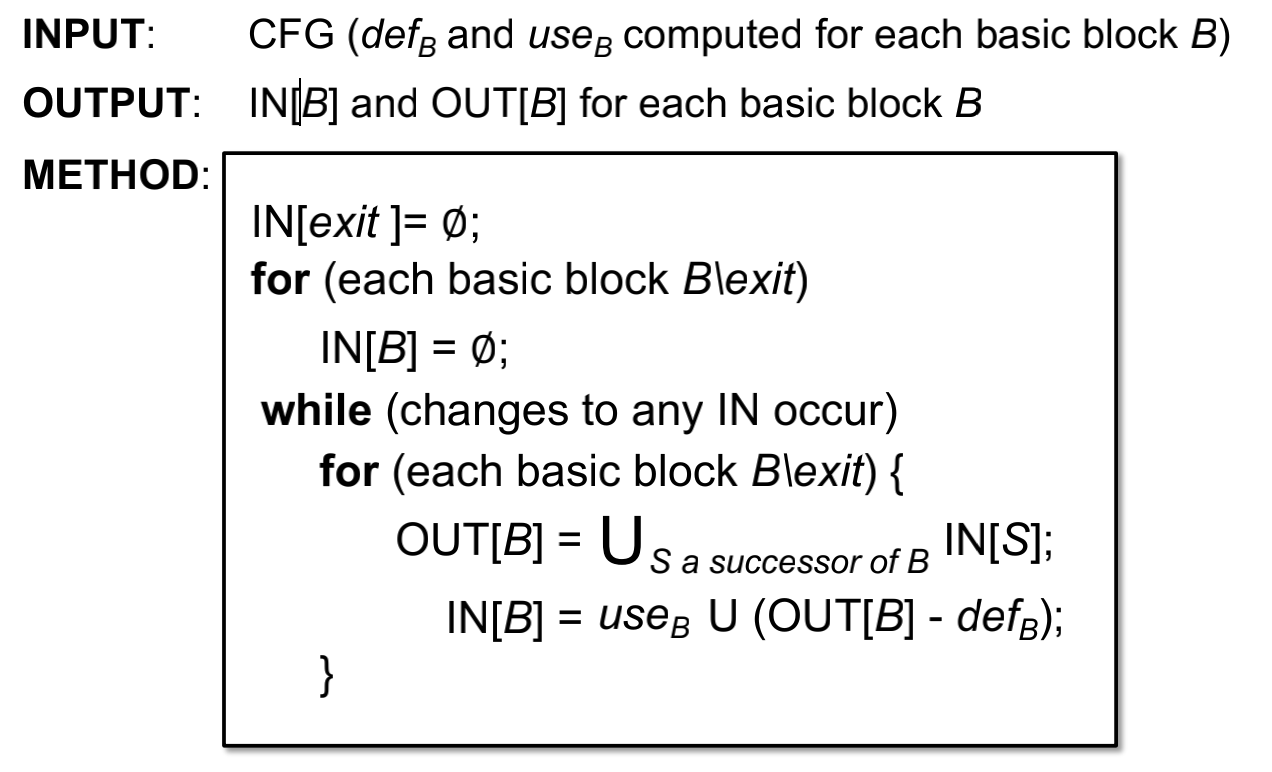Example: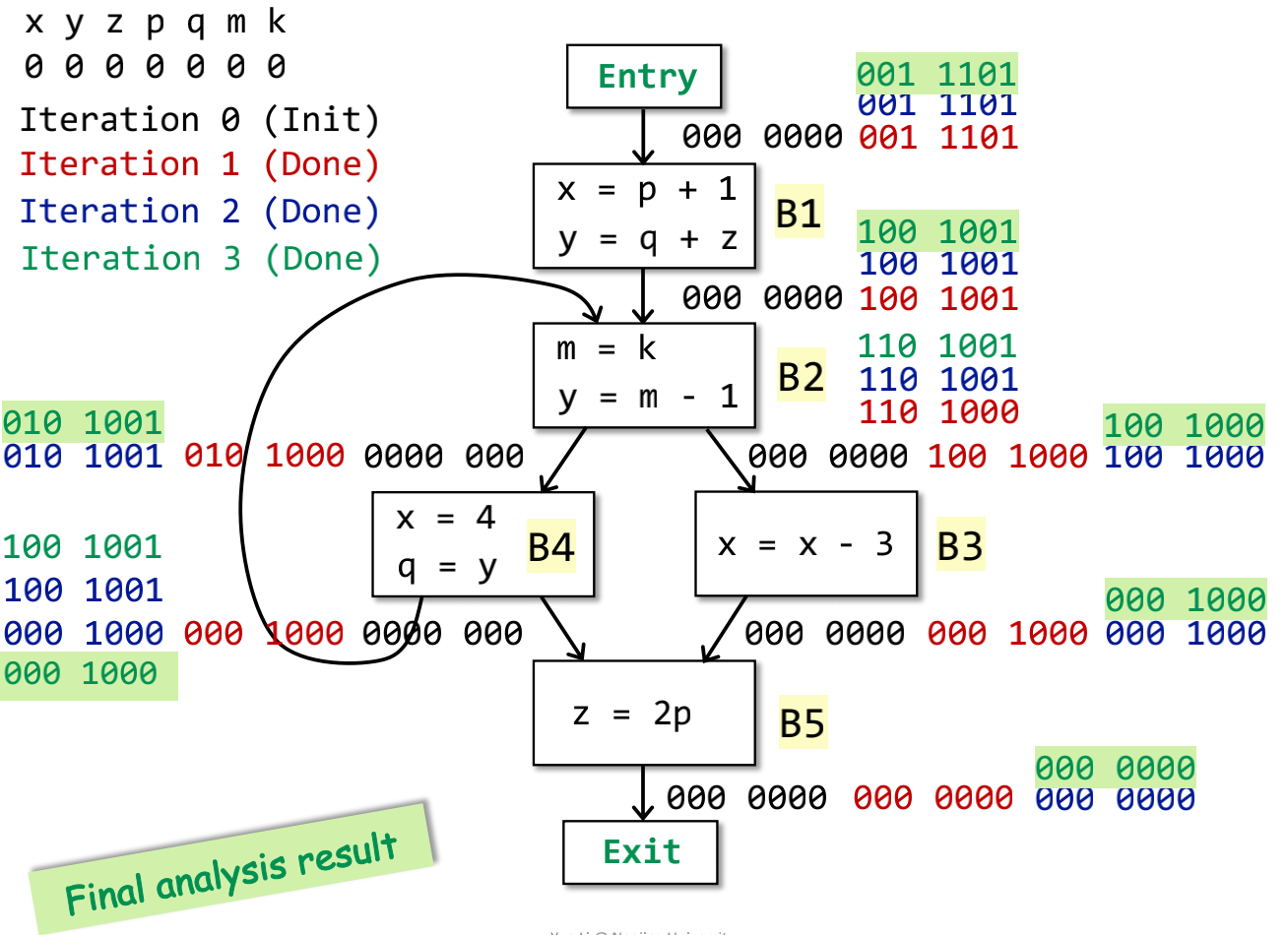##### Available Expressions Analysis

An expression x op y is available at program point p if all paths from the entry to p must pass through the evaluation of x op y, and after the last evaluation of x op y, there is no redefinition of x or y

Algorithm: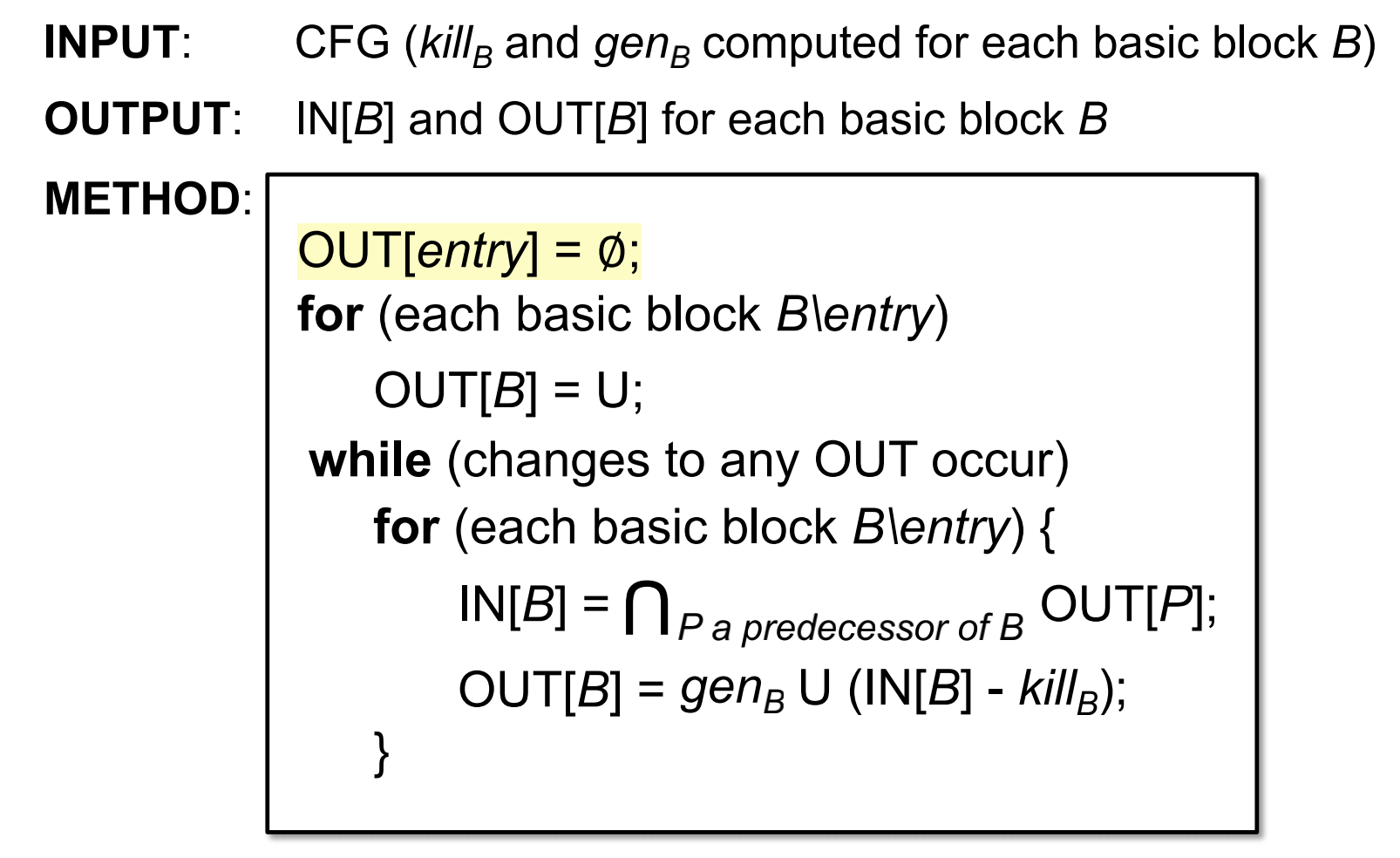Example: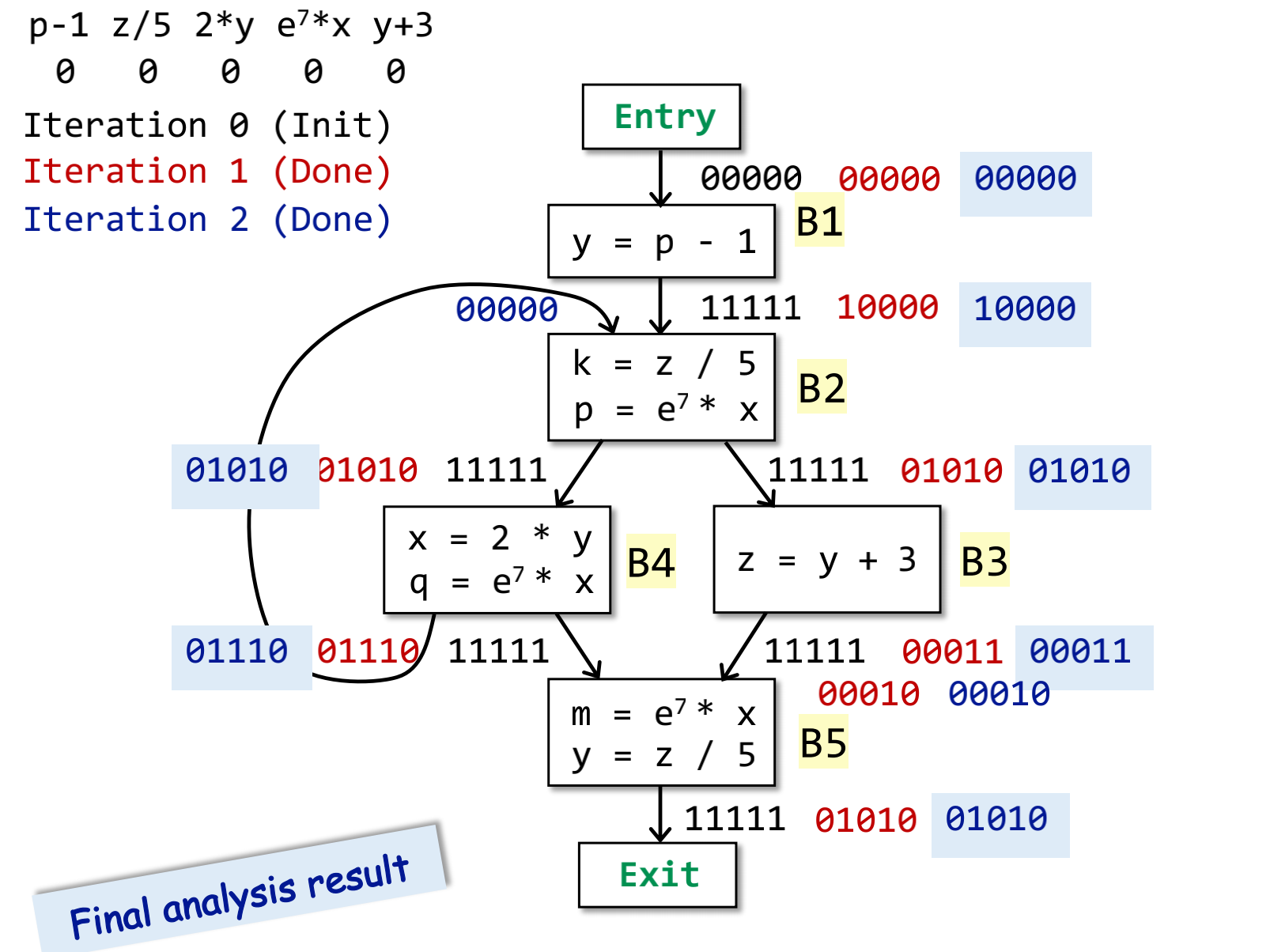##### Analysis Comparison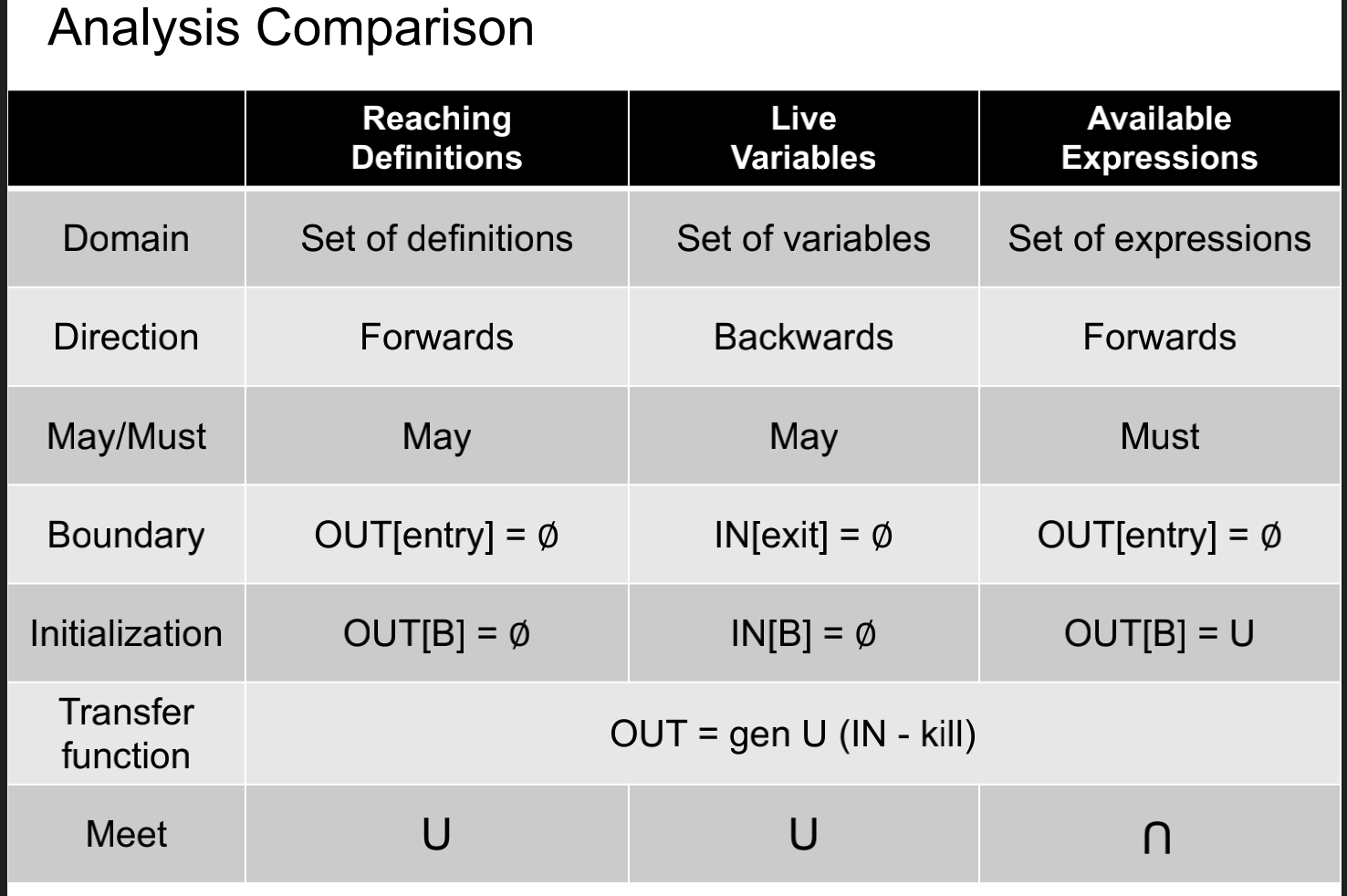#### Foundations

##### Partial Order

We define poset as a pari (P, ⊑) where ⊑ is a binary relation that defines a partial ordering over P and ⊑ has the following properties:

Reflexivity(自反性)：∀x ∈ P, x ⊑ x

Antisymmetry(反对称性): ∀x, y ∈ P, x ⊑ y ∧ y ⊑ x ⟹ x = y

Transitivity(传递性): ∀x, y, z ∈ P, x ⊑ y ∧ y ⊑ z ⟹ x ⊑ z

Partial means for a pair of set elements in P,they could be incomparable; in other words, not necessary that every pair of set elements must satisfy the ordeing ⊑

##### Upper and Lower Bounds

Given a poset (P, ⊑) and its subset S that S ⊆ P, we say that

u ∈ P is an upper bound of S, if ∀x ∈ S, x ⊑ u. Similarly,

l ∈ P is an lower bound of S, if ∀x ∈ S, l ⊑ x.

We define the least upper bound(lub or join) of S, written ⊔S, if for every upper bound of S, say u, ⊔S ⊑ u.

Similarly, We define the greatest lower bound (glb or meet) of S, written ⊓S, is for every lower bound of S,say l, l ⊑ ⊓S.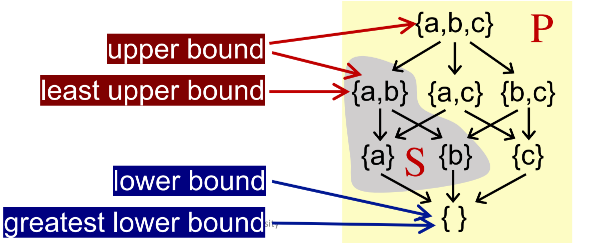Some Properties:

• Not every poset has lub or glb

• But if a poset has lub or glb, it will be unique

##### Lattice

Given a poset (P, ⊑), ∀a, b ∈ P, if a ⊔ b and a ⊓ b exist, then (P, ⊑) is called a lattice

(A poset is a lattice if every pair of its elements has a least upper bound and a greatest lower bound)###

##### Complete Lattice

All subsets of a lattice have a least upper bound and a greatest lower bound

Every finite lattice is a complete lattice. But complete lattice is not necessarily finite

##### Product Lattice

Given lattices L1 = (P 1 , ⊑1 ), L2 = (P 2 , ⊑2 ), ..., Ln = (P n , ⊑n ), if for all i,(P i , ⊑i ) has ⊔i (least upper bound) and ⊓i (greatest lower bound), then we can have a product lattice Ln = (P, ⊑) that is defined by: • P = P 1 × ... × P n • (x 1 , ..., xn ) ⊑ (y 1 , ..., yn ) ⟺ (x 1 ⊑ y1 ) ∧ ... ∧ (xn ⊑ yn ) • (x 1 , ..., xn ) ⊔ (y 1 , ..., yn ) = (x 1 ⊔1 y1 , ..., xn ⊔n yn ) • (x 1 , ..., xn ) ⊓ (y 1 , ..., yn ) = (x 1 ⊓1 y1 , ..., xn ⊓n yn )

A product lattice is a lattice

If a product lattice L is a product of complete lattices,then L is also complete

##### Fixed-Point Theorem

Given a complete lattice(L,⊑) if

1. f: L -> L is monotonic (单调)

2. L is finite (有界)

then the least fixed point of f can be found by iterating f(⊥), f(f(⊥)), ..., f (... (⊥)...) until a fixed point is reached

and the greatest fixed point of f can be found by iterating f(T), f(f(T)), ......, f(...f (T )...) until a fixed point is reached